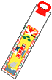\$B<78^;0(B (\$B\$7\$A(B - \$B\$4(B - \$B\$5\$s(B) - 11\$B7n(B15\$BF|(B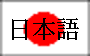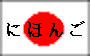\$B!A(BA gala day for children of three, five and seven years of age\$B!A(B

11\$B7n(B15\$BF|\$O<78^;0\$G!"<7:M\$H8^:M\$H;0:M\$N;R\$I\$b\$?\$A\$O\$-\$l\$\$\$JCeJ*\$rCe\$F!"(B \$BN>?F\$H\$\$\$C\$7\$g\$K?@\$B;2\$j(B\$B\$7\$^\$9!#F|K\\$G\$O(B\$B4q?t(B\$B\$O\$*\$a\$G\$?\$\$?t\$G!";R\$I\$b\$N4q?t\$NG/!JCK\$N;R\$O;0:M(B \$B\$H8^:M!"=w\$N;R\$O;0:M\$H<7:M!K\$K!"(B\$B7r9/(B\$B\$H(B\$B9,\$;(B\$B\$r4j\$C\$F(B\$B\$*=K\$\$(B\$B\$r\$7\$^\$9!#?@(B \$B\$B@i:P0;(B\$B\$r\$\$\$?\$@\$\$\$F5"\$j\$^\$9!#(B \$B@i:P0;\$O@V\$HGr\$N(B\$B:YD9\$\$(B\$B0;\$G!"(B\$BDa(B \$B\$H(B\$B55(B\$B\$N3(\$N\$D\$\$\$?(B\$BB^(B\$B\$KF~\$C(B \$B\$F\$\$\$^\$9!#@i:P\$H\$\$\$&\$N\$O!V@iG/!W\$H\$\$\$&0UL#\$G\$9!#@N\$+\$i!VDa\$O@iG/!"55\$O(B \$BK|G/!W\$H8@\$o\$l\$FF|K\\$G\$ODa\$H55\$O(B\$BD9@8\$-(B\$B\$N%7%s%\%k(B \$B\$J\$N\$G\$9!#(B

Vocabulary Notes:
\$B;2\$k(B		\$B\$^\$\$\$k(B		to visit [worship at] a temple or shrine
\$B4q?t(B		\$B\$-\$9\$&(B		odd numbers
\$B7r9/(B		\$B\$1\$s\$3\$&(B	health
\$B9,\$;(B		\$B\$7\$"\$o\$;(B	happiness
(\$B\$*(B)\$B=K\$\$(B	(\$B\$*(B)\$B\$\$\$o\$\$(B	celebration
\$B@i:P0;(B		\$B\$A\$H\$;\$"\$a(B	thousand-year candy
\$B:YD9\$\$(B		\$B\$[\$=\$J\$,\$\$(B	long and narrow
\$BDa(B		\$B\$D\$k(B		crane
\$B55(B		\$B\$+\$a(B		turtle
\$BB^(B		\$B\$U\$/\$m(B		bag
\$BD9@8\$-(B		\$B\$J\$,\$\$\$-(B	longevity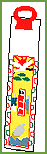\$B@i:P0;(B
\$B@i:P0;\$O!":YD9\$\$!"@V\$HGr\$N%-%c%s%G%#!<\$G!"Da\$H55\$NIA\$+\$l\$?;fB^\$KF~\$C(B \$B\$F\$\$\$^\$9!#(B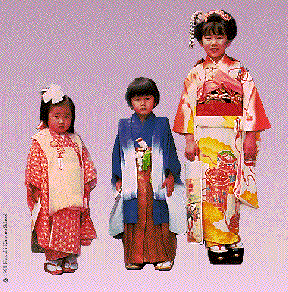3\$B:M\$N=w\$N;R!"(B5\$B:M\$NCK\$N;R!"(B7\$B:M\$N=w\$N;R(B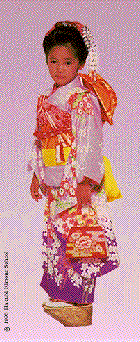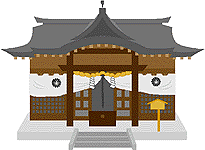\$B?@\$B?@